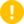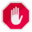## Name

ST_Intersection — (T) Returns a geometry that represents the shared portion of geomA and geomB.

## Synopsis

`geometry ST_Intersection(` geometry geomA , geometry geomB `)`;

`geography ST_Intersection(` geography geogA , geography geogB `)`;

## Description

Returns a geometry that represents the point set intersection of the Geometries.

In other words - that portion of geometry A and geometry B that is shared between the two geometries.

If the geometries do not share any space (are disjoint), then an empty geometry collection is returned.

ST_Intersection in conjunction with ST_Intersects is very useful for clipping geometries such as in bounding box, buffer, region queries where you only want to return that portion of a geometry that sits in a country or region of interest.Geography: For geography this is really a thin wrapper around the geometry implementation. It first determines the best SRID that fits the bounding box of the 2 geography objects (if geography objects are within one half zone UTM but not same UTM will pick one of those) (favoring UTM or Lambert Azimuthal Equal Area (LAEA) north/south pole, and falling back on mercator in worst case scenario) and then intersection in that best fit planar spatial ref and retransforms back to WGS84 geography.Do not call with a `GEOMETRYCOLLECTION` as an argumentThis function will drop the M coordinate values if present.If working with 3D geometries, you may want to use SFGCAL based ST_3DIntersection which does a proper 3D intersection for 3D geometries. Although this function works with Z-coordinate, it does an averaging of Z-Coordinate values when `postgis.backend=geos`. `postgis.backend=sfcgal`, it will return a 2D geometry regardless ignoring the Z-Coordinate. Refer to postgis.backend for details.

Performed by the GEOS moduleThis method is also provided by SFCGAL backend.

Availability: 1.5 support for geography data type was introduced.This method implements the OpenGIS Simple Features Implementation Specification for SQL 1.1. s2.1.1.3This method implements the SQL/MM specification. SQL-MM 3: 5.1.18

## Examples

```SELECT ST_AsText(ST_Intersection('POINT(0 0)'::geometry, 'LINESTRING ( 2 0, 0 2 )'::geometry));
st_astext
---------------
GEOMETRYCOLLECTION EMPTY
(1 row)
SELECT ST_AsText(ST_Intersection('POINT(0 0)'::geometry, 'LINESTRING ( 0 0, 0 2 )'::geometry));
st_astext
---------------
POINT(0 0)
(1 row)

---Clip all lines (trails) by country (here we assume country geom are POLYGON or MULTIPOLYGONS)
-- NOTE: we are only keeping intersections that result in a LINESTRING or MULTILINESTRING because we don't
-- care about trails that just share a point
-- the dump is needed to expand a geometry collection into individual single MULT* parts
-- the below is fairly generic and will work for polys, etc. by just changing the where clause
SELECT clipped.gid, clipped.f_name, clipped_geom
FROM (SELECT trails.gid, trails.f_name, (ST_Dump(ST_Intersection(country.the_geom, trails.the_geom))).geom As clipped_geom
FROM country
INNER JOIN trails
ON ST_Intersects(country.the_geom, trails.the_geom))  As clipped
WHERE ST_Dimension(clipped.clipped_geom) = 1 ;

--For polys e.g. polygon landmarks, you can also use the sometimes faster hack that buffering anything by 0.0
-- except a polygon results in an empty geometry collection
--(so a geometry collection containing polys, lines and points)
-- buffered by 0.0 would only leave the polygons and dissolve the collection shell
SELECT poly.gid,  ST_Multi(ST_Buffer(
ST_Intersection(country.the_geom, poly.the_geom),
0.0)
) As clipped_geom
FROM country
INNER JOIN poly
ON ST_Intersects(country.the_geom, poly.the_geom)
WHERE Not ST_IsEmpty(ST_Buffer(ST_Intersection(country.the_geom, poly.the_geom),0.0));
```

## Examples: 2.5Dish

Geos is the default backend if not set. Note this is not a true intersection, compare to the same example using ST_3DIntersection.

```set postgis.backend=geos;
select ST_AsText(ST_Intersection(linestring, polygon)) As wkt
from  ST_GeomFromText('LINESTRING Z (2 2 6,1.5 1.5 7,1 1 8,0.5 0.5 8,0 0 10)') AS linestring
CROSS JOIN ST_GeomFromText('POLYGON((0 0 8, 0 1 8, 1 1 8, 1 0 8, 0 0 8))') AS polygon;

st_astext
---------------------------------------
LINESTRING Z (1 1 8,0.5 0.5 8,0 0 10)
```

If your PostGIS is compiled with sfcgal support, have option of using sfcgal, but note if basically cases down both geometries to 2D before doing intersection and returns the ST_Force2D equivalent result which is a 2D geometry

```set postgis.backend=sfcgal;
select ST_AsText(ST_Intersection(linestring, polygon)) As wkt
from  ST_GeomFromText('LINESTRING Z (2 2 6,1.5 1.5 7,1 1 8,0.5 0.5 8,0 0 10)') AS linestring
CROSS JOIN ST_GeomFromText('POLYGON((0 0 8, 0 1 8, 1 1 8, 1 0 8, 0 0 8))') AS polygon;

wkt
----------------------------------------------
MULTILINESTRING((0.5 0.5,0 0),(1 1,0.5 0.5))
```